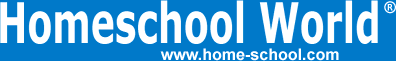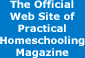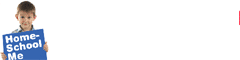Home Curriculum Catalog Articles Contests Events Groups Forum News ContactGetting Started Preschool/K Elementary Middle School High School College & Career Prep Special Needs Group Activities Research FAQs# Lies, Part I: The Importance of Consistent Verbalizations

By Bob Hazen
Printed in Practical Homeschooling #17, 1997.Why three and five does not make eight.The previous article in this series dealt with the importance of using multiple models (Real, Musical, Verbal, Concrete, Pictorial, Abstract, and Written) to represent mathematical ideas, facts, and procedures. This article focuses specifically on the careful use of the Verbal model to explain math in ways that are both accurate (fully true) and consistent (true across grades and topics). Verbalizations are translations into or from the Verbal mode, whether paired with Abstract symbols, Concrete manipulatives, or Pictorial sketches.

Simply stated, too often certain mathematical verbalizations are lies. By lies, I mean statements that are simply wrong, or only true within a very limited context. Our verbal explanations need to be both accurate and consistent: accurate to the mathematical facts, concepts, and activities they are describing; and also consistent both with other modalities and with other math topics in later grades.

Perhaps the best way to illustrate the importance of accurate and consistent verbalizations is to examine some of the lies that are often made when math is explained to elementary students.

Example #1

The Lie: With the subtraction exercise 752 - 196, children often hear "In the ones' place, you can't take 6 from 2, so we need to borrow . . ."

The Problem: It's true: if you only have 2 things, how can you possibly take 6 away? But this is only verbalizing the "physical removal" view of subtraction; and it is not the best way to consistently explain subtraction, because it only works if you are taking away less than what you started with. A broader explanation - one that is more accurate and consistent - is needed.

The Why: We can take 6 from 2. It happens when the temperature drops 60 degrees, from 20° to -40°, or when you have a checkbook balance of only \$2 and write a check for \$6, ending with a balance of -\$4 (before the bank charges you for the overdraft). Even children in K-1 have some experiential sense of negative numbers, such as card games (where a negative amount is called "going in the hole") or owing money ("I have 2 pennies but I owe Dad 6 pennies, so after I give him my 2 pennies, I'll still owe him 4 pennies").

The Confusion Now: With the exercise 752 - 196, children who consistently hear "You can't take 6 from 2" often conclude erroneously (but somewhat logically) that they should just take 2 from 6. A common error with subtraction is that students think they should take the smaller number (whether on top or bottom) from the larger number (again, whether on top or bottom), not realizing that subtraction is always supposed to be the top number minus the bottom number.

The Confusion Later: At the junior-high level, students are introduced to negative numbers and are specifically taught to calculate that "2 - 6 = -4." You might have to imagine their confusion if they have heard for years, "You can't take 6 from 2 . . . ." But as a junior-high teacher, I've seen this confusion far too often.

The Truth: A truthful explanation for 752 - 196 is, "In the ones' column, 2 minus 6 will give a negative number, which won't be helpful here, so we have to regroup [borrow] in the tens' column." For an exercise with regrouping in the tens' column, like 853 - 271, we could say, "In the tens' column, 5 tens minus 7 tens will give a negative amount of tens, which won't help us here, so we have to regroup [borrow] in the hundreds' column." A truthful explanation like this - a consistent, accurate verbalization - helps set kids up for success when working with negative numbers in later years.

Example #2

The Lie: The addition exercise 3 + 5 = 8 is often verbalized as "Three and five is eight."

The Problem: This verbalization implies that "+" means "and," and therefore implies that "and" means "+."

The Why: Grammatically, "and" is a conjunction, not a verb, so it should show no action at all. "And" is used in too many situations in life and math to be associated with the specific operation of addition.

The Confusion Now: The problem here is that 3 and 5 equal 8 if we are adding; but 3 and 5 equal 15 if we multiply, while 3 and 5 equal -2 if we subtract, and 3 and 5 equal a fraction (3/5) if we divide.

The Confusion Later: A commonly worded problem in grades 5-8 is, "Find the product of 3 and 5," and the answer is 15. However, I have worked with students who for years have heard that "and" means "plus," so in their minds, "Find the product of 3 and 5" means "Find the product of 3 plus 5." What in the world does that mean? "Product" means multiply, but "plus" means add, so what is the student supposed to do?

The Truth: Since "and" is not a verb but a conjunction, let's only use "and" when the desired action is already specified in some other way. Some truthful verbalizations for 3 + 5 include "three plus five," "three added to five," "three joined with five," "three combined with five," or even "the sum of three and five." In the last verbalization, the use of "and" is proper, because the term "sum" specifies that we are to add the two numbers that follow the word "of" - that is, the 3 should be added with the 5.

Example #3

The Lie: The addition exercise 3 + 5 = 8 is often verbalized as "Three plus five makes eight."

The Problem: Verbalizing an equals sign as "makes" implies that an action has occurred. If we think of the symbols "3 + 5 = 8" as a sentence, then the equal sign is the verb - but it is not an action verb. "Equals" is a stative verb, a verb of state or being, like "is."

The Why: The action in "3 + 5 = 8" is not in the equal sign; it's in the plus sign. The "+" tells us what to do: join the amount of 3 with the amount of 5.

The Confusion Now: Students who hear the "=" symbol translated into "makes" get the idea that the symbol means "do something" or "the answer is . . . ."

The Confusion Later: In secondary math, students encounter a variety of statements in which there is "no answer" as such. Algebra and later courses are peppered with statements using the = sign that stress ideas, not numerical "answers." One profound idea using an equal sign is simply that the two sides of an equation merely have the same value, such as the Distributive Property [that a(b + c) = ab + ac, and that ab + ac = a(b + c)], or the Pythagorean theorem [that the sides of a right triangle are related by a2 + b2 = c2]. Thinking of "=" as "makes" is a stumbling block.

The Truth: The best, most consistent verbal translation of the "=" sign is simply "is" - "3 + 5 is 8." Another good translation is "3 + 5 is the same value as 8." The fundamental meaning of the symbol "=" is "sameness" or "same value"; nothing more, literally, and also nothing less.

Consistent verbalizations connect with learning styles. Accurate verbalizations are a key factor in creating a high degree of consistency between what students say, see, build, write, draw, and even sing [see "Why Manipulatives Are Not Enough," PHS #16]. This "inter-modal" consistency conveys to children that math is a sensible experience. But for math teaching to really be "say what you see, write what you say," there must first be a high degree of consistency between what is seen and said and written. Accurate, consistent verbalizations make that connection stronger.Terms of Use   Privacy Policy Copyright ©1993-2023 Home Life, Inc.